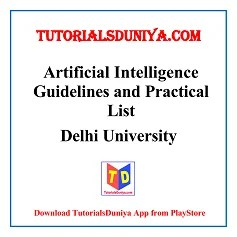• ## Artificial Intelligence Guidelines and Practical List

Course Objective: This course introduces the basic concepts and techniques of Artificial Intelligence (AI). The course aims to introduce intelligent agents and reasoning, heuristic search techniques, game playing, knowledge representation, reasoning with uncertain knowledge.

### Artificial Intelligence Guidelines

Introduction: Introduction to artificial intelligence, background and applications, Turing test, rational agents, intelligent agents, structure, behaviour and environment of intelligent agents.

Knowledge Representation: Propositional logic, first order predicate logic, resolution principle, unification, semantic nets, conceptual dependencies, frames, scripts, production rules, conceptual graphs.

Reasoning with Uncertain Knowledge: Uncertainty, non-monotonic reasoning, truth maintenance systems, default reasoning and closed world assumption, Introduction to probabilistic reasoning, Bayesian probabilistic inference, introduction to fuzzy sets and fuzzy logic, reasoning using fuzzy logic.

Problem Solving and Searching Techniques: Problem characteristics, production systems, control strategies, breadth first search, depth first search, hill climbing and its variations, heuristics search techniques: best first search, A* algorithm, constraint satisfaction problem, means-end analysis.

Game Playing: introduction to game playing, min-max and alpha-beta pruning algorithms.

Prolog Programming: Introduction to Programming in Logic (PROLOG), Lists, Operators, basic Input and Output.

Understanding Natural Languages: Overview of linguistics, Chomsky hierarchy of grammars, parsing techniques.

Ethics in AI, Fairness in AI, Legal perspective

Artificial Intelligence Reference Books:

1. Rich, E. & Knight,K. (2012). Artificial Intelligence. 3rd edition. Tata McGraw Hill. Pearson Education

2. Russell, S.J. & Norvig, P. (2015) Artificial Intelligence – A Modern Approach. 3rd edition.

### Artificial Intelligence Practicals List

1. Write a prolog program to calculate the sum of two numbers.
2. Write a Prolog program to implement max(X, Y, M) so that M is the maximum of two numbers X and Y.
3. Write a program in PROLOG to implement factorial (N, F) where F represents the factorial of a number N.
4. Write a program in PROLOG to implement generate_fib(N,T) where T represents the Nth term of the fibonacci series.
5. Write a Prolog program to implement GCD of two numbers.
6. Write a Prolog program to implement power (Num,Pow, Ans) : where Num is raised to the power Pow to get Ans.
7. Prolog program to implement multi (N1, N2, R) : where N1 and N2 denotes the numbers to be multiplied and R represents the result.
8. Write a Prolog program to implement memb(X, L): to check whether X is a member of L or not.

9. Write a Prolog program to implement conc (L1, L2, L3) where L2 is the list to be appended with L1 to get the resulted list L3.
10. Write a Prolog program to implement reverse (L, R) where List L is original and List R is reversed list.
11. Write a program in PROLOG to implement palindrome (L) which checks whether a list Lis a palindrome or not.
12. Write a Prolog program to implement sumlist(L, S) so that S is the sum of a given list L.
13. Write a Prolog program to implement two predicates evenlength(List) and oddlength(List) so that they are true if their argument is a list of even or odd length respectively.
14. Write a Prolog program to implement nth_element (N, L, X) where N is the desired position, L is a list and X represents the Nth element of L.
15. Write a Prolog program to implement maxlist(L, M) so that M is the maximum number in the list.
16. Write a prolog program to implement insert_nth (I, N, L, R) that inserts an item I into Nth position of list L to generate a list R.
17. Write a Prolog program to implement delete_nth (N, L, R) that removes the element on Nth position from a list L to generate a list R.
18. Write a program in PROLOG to implement merge (L1, L2, L3) where L1 is first ordered list and L2 is second ordered list and L3 represents the merged list.

Source: https://www.du.ac.inArtificial Intelligence Guidelines and Programs List
Delhi University (DU)Artificial Intelligence Compiled Book
Delhi University (DU)

TutorialsDuniya.com is a witty content portal that is optimized for college students which mainly focuses on Computer Science Subjects.
Our aim to provide easily accessible and well organised quality content to all the students.